# Ex.3.1 Q6 Understanding Quadrilaterals Solution - NCERT Maths Class 8

Go back to  'Ex.3.1'

## Question

Find the angle measure $$x$$ in the following figures: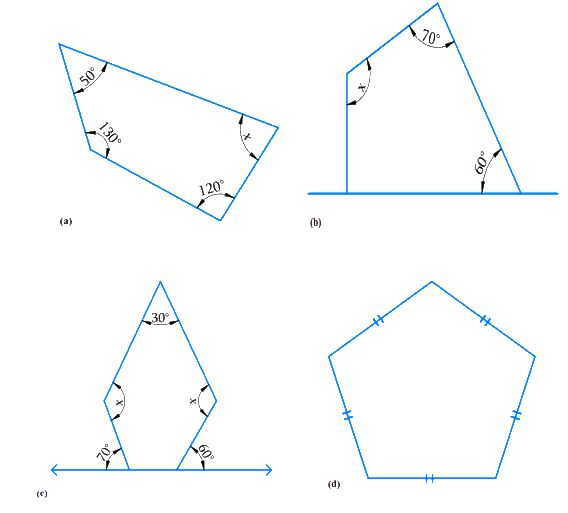## Text Solution

What is Known?

Sum of the measures of all interior angles of a quadrilateral is $$360^\circ$$ and that of a pentagon is $$540^\circ$$

What is Unknown?

Angle $$x$$ in the above figures  $$a, b, c$$ and $$d$$

Steps: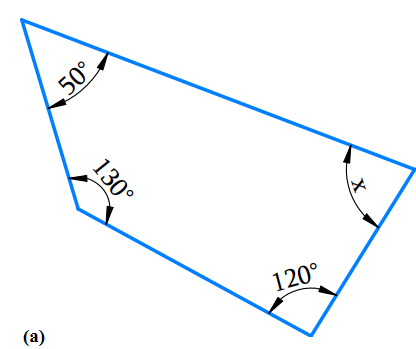a) The above figure has $$4$$ sides and hence it is a quadrilateral.

Using the angle sum property of a quadrilateral,

\begin{align}{{50}^{\rm{o}}} + {{130}^{\rm{o}}} + {{120}^{\rm{o}}} + x &= {{360}^{\rm{o}}}\\ {{300}^{\rm{o}}} + x &= {{360}^{\rm{o}}}\\x & = {{360}^{\rm{o}}} - {{300}^{\rm{o}}}\\x &= {{60}^{\rm{o}}} \end{align}

b) Using the angle sum property of a quadrilateral.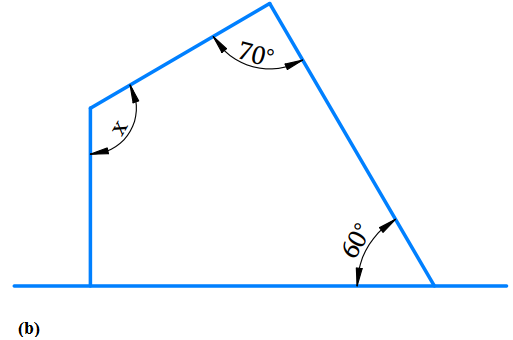\begin{align}{{90}^\circ } + {{60}^\circ } + {{70}^\circ } + x& = {{360}^\circ }\\{{220}^\circ } + x &= {{360}^\circ }\\{220^\circ } + x &= {360^\circ }\\x& = {360^\circ } - {220^\circ }\\x &= {140^\circ }\end{align}

c) The given figure is a pentagon $$(n=5)$$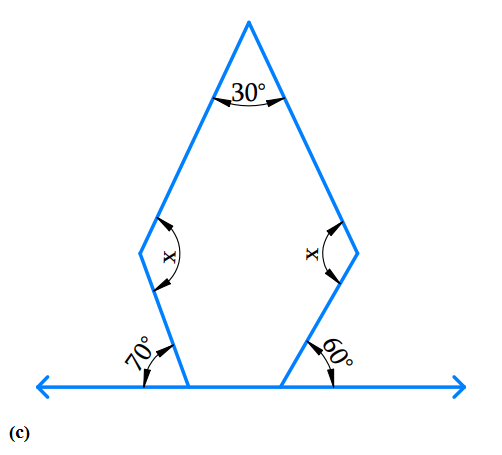Angle sum of a polygon

\begin{align}& = ({\rm{n} - 2) \times {{180}^{\rm{o}}}}\\&=(5 - 2) \times {{180}^{\rm{o}}}\\& =3 \times {{180}^{\rm{o}}}\\ & = {540}^{\rm{o}}\end{align}

Sum of the interior angle of pentagon is $$540^\circ$$

Angles at the bottom are linear pair.

$$\therefore$$ First base interior angle a

\begin{align}& = {180^{\rm{o}}} - {70^{\rm{o}}}{\rm{ }}\left( \begin{array}{l}{\text{angle of straight }}\\{\text{line is }}{180^{\rm{o}}}\end{array} \right)\\ &= {\mkern 1mu} {\mkern 1mu} {110^{\rm{o}}} \end{align}

$$\therefore$$  Second base interior angle b

\begin{align}&= {{180}^{\rm{o}} - {{60}^{\rm{o}}}}\\& = {{120}^{\rm{o}}}\end{align}

Angle sum of a polygon $$= ({\rm{n}} - 2) \times {180^o}$$

\begin{align}\\{30^o} + x + {110^o} + {120^o} + x &= {540^o}\\2x + {260^{\rm{o}}}\, &= {540^{\rm{o}}}\\2x &\!=\!{540^{\rm{o}}}\!-\!{260^{\rm{o}}}\\2x &= {280^{\rm{o}}}\\x &= \frac{{{{280}^{\rm{o}}}}}{2}\\x &= {140^{\rm{o}}}\end{align}

D) The given figure is pentagon $$(n-5)$$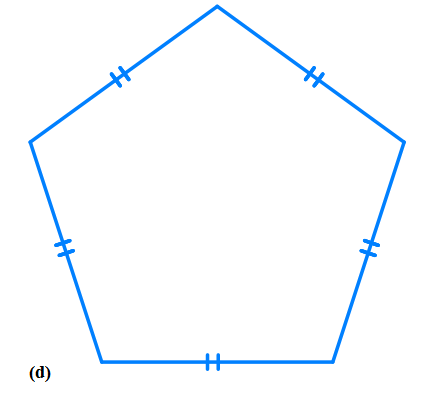Angle sum of a polygon

\begin{align}& = ({\rm{n}} - 2) \times {{180}^{\rm{o}}}\\& = (5 - 2) \times {{180}^{\rm{o}}}\\& = 3 \times {{180}^{\rm{o}}}\\& = {{540}^{\rm{o}}}\end{align}

Sum of the interior angle of pentagon is $$540^\circ$$

Angle sum of a polygon

\begin{align}&= {x + x + x + x + x = {{540}^{\rm{o}}}}\\{\rm{ i}}{\rm{.e}}{\rm{. }}\ \qquad 5x &= {{540}^{\rm{o}}}\\x &= {\frac{{{{540}^{\rm{o}}}}}{5}}\\x & = {{108}^{\rm{o}}}\end{align}

A pentagon is a regular polygon i.e. it is both ‘equilateral’ and ‘equiangular’. Thus, the measure of each interior angle of the pentagon are equal.

Hence each interior angle is $$108^\circ .$$

Learn from the best math teachers and top your exams

• Live one on one classroom and doubt clearing
• Practice worksheets in and after class for conceptual clarity
• Personalized curriculum to keep up with school